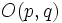# Pseudo-Riemannian manifold

This article defines a differential manifold with the following additional structure -- the structure group is reduced to: pseudo-orthogonal group viz a group of the form$O(p,q)$

## Definition

A pseudo-Riemannian manifold or semi-Riemannian manifold is the following data:

• A differential manifold$M$
• A symmetric nondegenerate bilinear form of type$(p,q)$ for fixed integers$p$ and$q$, on the tangent space at each point, such that the form varies smoothly with the point.

In other words, a pseudo-Riemannian manifold is a reduction of the structure group to$O(p,q)$ on a differential manifold.

## Parallel with Riemannian manifolds

Many of the constructions/quantities that we can associate with a Riemannian manifold, we can also associate with a pseudo-Riemannian manifold.

### Levi-Civita connection

Further information: Levi-Civita connection for a pseudo-Riemannian manifold

Any pseudo-Riemannian manifold has a unique Levi-Civita connection. The notion of Levi-Civita connection is defined here in the same way as for a Riemannian manifold: the Levi-Civita connection must be compatible with the bilinear form at every point, and it must be torsion-free.

The proof is exactly the same as for a Riemannian manifold, because the only thing we used about the bilinear form was its nondegeneracy: we used that to say that knowing the inner product with every vector can lead us to recover the original vector.

### Riemann curvature tensor

Further information: Riemann curvature tensor for a pseudo-Riemannian manifold

For any connection on a differential manifold, we have an associated Riemann curvature tensor. Thus, for a pseudo-Riemannian manifold, we can look at the Riemann curvature tensor obtained corresponding to the Levi-Civita connection.

We can also define the corresponding 4-tensor using the inner product.

### Ricci curvature tensor

Further information: Ricci curvature tensor for a pseudo-Riemannian manifold

The Ricci curvature tensor for a Riemannian manifold is defined as the trace of the Riemann curvature tensor when viewed as a map on the second and fourth variable, fixing the first and third variables. The Ricci curvature tensor is defined similarly for a pseudo-Riemannian manifold.